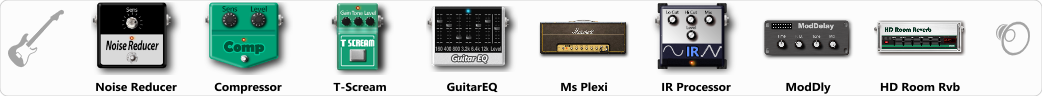# test

Discussion in 'ToneLib-GFX presets' started by dany1973, Mar 17, 2022.

1. test

Preset name: AC DC

Effects chain:Effect: "Noise Reducer" (Dynamics / Filter), active - "yes"
"Sens" = 41
"Mode" = Soft

Effect: "Compressor" (Dynamics / Filter), active - "yes"
"Sense" = 41
"Level" = 41

Effect: "T-Scream" (Overdrive / Distortion), active - "yes"
"Drive" = 52
"Tone" = 56
"Level" = 69

Effect: "GuitarEQ" (Dynamics / Filter), active - "yes"
"160 Hz" = 1
"400 Hz" = 2
"800 Hz" = 4
"1.6 kHz" = 5
"3.2 kHz" = 3
"6.4 kHz" = 2
"12 kHz" = 1
"Level (dB)" = 4

Effect: "Ms Plexi" (Amp simulators), active - "yes"
"Gain" = 50
"Bass" = 40
"Middle" = 60
"Treble" = 42
"Presence" = 32
"Master" = 100
"Output" = 50
"Level (dB)" = 9

Effect: "IR Processor" (Dynamics / Filter), active - "yes"
"IR" = marshall-2_impact
"Low Cut (Hz)" = 0
"Hi Cut (kHz)" = 20.0
"Mix" = 100
"Level (dB)" = 0

Effect: "ModDly" (Delay), active - "yes"
"Time" = 521
"Feedback" = 25
"Tone" = 56
"Speed" = 1.6
"Mix" = 61

Effect: "HD Room Rvb" (Reverb), active - "yes"
"Time" = 3.9
"PreLPF" = 77
"PreDelay" = 104
"HiDamp" = 65
"LoGain" = -3.5
"Mix" = 33

Note: You will need to download and install the ToneLib-GFX software to use the preset.

#### Attached Files:

• ###### test.tlgfx
File size:
19.4 KB
Views:
1,915
Skeve Nelis likes this.